# Ellipse facts for kids

Kids Encyclopedia Facts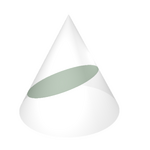An ellipse obtained as the intersection of a cone with a plane.

An ellipse is a shape that looks like an oval or a flattened circle.

In geometry, an ellipse is a plane curve which results from the intersection of a cone by a plane in a way that produces a closed curve.

Circles are special cases of ellipses, obtained when the cutting plane is perpendicular to the cone's axis. An ellipse is also the locus of all points of the plane whose distances to two fixed points add to the same constant.

A circle has one center, called a focus, but an ellipse has two foci.

An ellipse is simply all points on a graph that the sum of the distances from 2 points are the same. For example, an ellipse can be made by putting two pins into cardboard and a circle of string around those two, then putting a pencil in the loop and pulling as far as possible without breaking the string in all directions. The orbits of the planets are ellipses, with the sun at one focus and nothing at the other.

The equation of an ellipse is :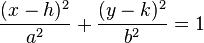$\frac{(x-h)^{2}}{a^{2}} + \frac{(y-k)^{2}}{b^{2}} = 1$
where the center of the ellipse is (h,k). 2A is the length from each end of the longer skinnier side. 2b is the length of the 2 ends of the short side. A²-B²=C² for c is the length between the foci and the center.

## Drawing ellipses

Ellipses appear in descriptive geometry as images (parallel or central projection) of circles. So it is essential to have tools to draw an ellipse. Nowadays the best tool is the computer. During the times before this tool was not available and one was restricted to compass and ruler for the construction of single points of an ellipse. But there are technical tools (ellipsographs) to draw an ellipse in a continuous way like a compass for drawing a circle, too. The principle of ellipsographs were known to Greek mathematicians (Archimedes, Proklos) already.

If there is no ellipsograph available, the best and quickest way to draw an ellipse is to draw an Approximation by the four osculating circles at the vertices.

For any method described below

• the knowledge of the axes and the semi-axes is necessary (or equivalent: the foci and the semi-major axis).

If this presumption is not fulfilled one has to know at least two conjugate diameters. With help of Rytz's construction the axes and semi-axes can be retrieved.

### Pins-and-string method

The characterization of an ellipse as the locus of points so that sum of the distances to the foci is constant leads to a method of drawing one using two drawing pins, a length of string, and a pencil. In this method, pins are pushed into the paper at two points, which become the ellipse's foci. A string tied at each end to the two pins and the tip of a pencil pulls the loop taut to form a triangle. The tip of the pencil then traces an ellipse if it is moved while keeping the string taut. Using two pegs and a rope, gardeners use this procedure to outline an elliptical flower bed—thus it is called the gardener's ellipse.

A similar method for drawing confocal ellipses with a closed string is due to the Irish bishop Charles Graves.

### Paper strip method

The following method relies on the parametric representation: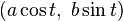$(a\cos t,\;b\sin t)$

This representation can be modeled technically by this simple method. The axes and semi axes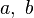$a,\; b$ have to be known.

Method

The first method starts with

• a strip of paper of length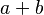$a+b$.

The point, where the semi axes meet is marked by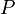$P$. If the strip slides with both ends on the axes of the desired ellipse, then point P traces the ellipse. For the proof one shows that point$P$ has the parametric representation$(a\cos t,\;b\sin t)$, where parameter$t$ is the angle of the slope of the paper strip.

A technichal realization of the motion of the paper strip can be achieved by a Tusi couple (s. animation). The device is able to draw any ellipse with a fixed sum$a+b$, which is the radius of the large circle. This restriction may be a disadvantage in real life. More flexible is the second paper strip method.

A nice application: If one stands somewhere in the middle of a ladder, which stands on a slippery ground and leans on a slippery wall, the ladder slides down and the persons feet trace an ellipse.

## Images for kidsEllipse Facts for Kids. Kiddle Encyclopedia.